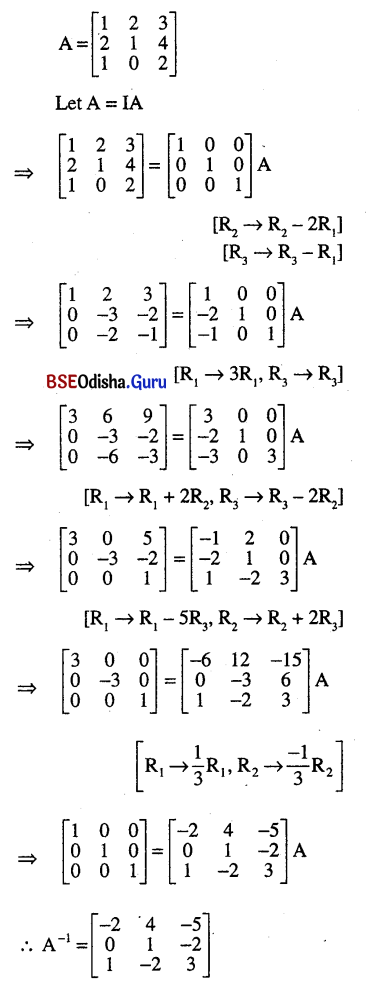# CHSE Odisha Class 12 Math Solutions Chapter 4 Matrices Ex 4(b)

Odisha State Board Elements of Mathematics Class 12 CHSE Odisha Solutions Chapter 4 Matrices Ex 4(b) Textbook Exercise Questions and Answers.

## CHSE Odisha Class 12 Math Solutions Chapter 4 Matrices Exercise 4(b)

Question 1.
State which of the following matrices are symmetric, skew-symmetric, both or not either: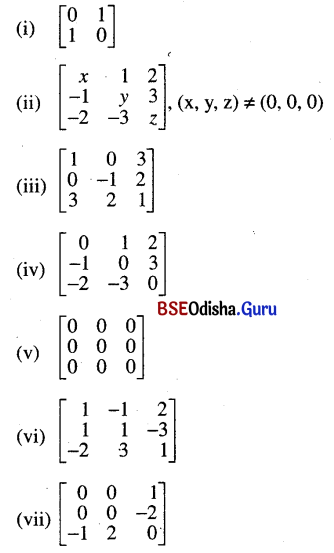Solution:
(i) Symmetric
(ii) Neither Symmetric nor skew-symmetric
(iii) Symmetric
(iv) Skew symmetric
(v) Both
(vi) Neither symmetric nor skew-symmetric
(vii) Skew symmetricQuestion 2.
State ‘True’ or ‘False’:
(i) If A and B are symmetric matrices of the same order and AB – BA ≠ 0, then AB is not symmetric.
Solution:
True

(ii) For any square matrix A, AA’ is symmetric.
Solution:
True

(iii) If A is any skew-symmetric matrix, then A2 is also skew-symmetric.
Solution:
False

(iv) If A is symmetric, then A2, A3, …, An are all symmetric.
Solution:
True

(v) If A is symmetric then A – A1 is both symmetric and skew-symmetric.
Solution:
False

(vi) For any square matrix (A – A1)2 is skew-symmetric.
Solution:
True

(vii) A matrix which is not symmetric is skew-symmetric.
Solution:
False

Question 3.
(i) If A and B are symmetric matrices of the same order with AB ≠ BA, final whether AB – BA is symmetric or skew symmetric.
Solution:
A and B are symmetric matrices;
Thus A’ = A and B’ = B
Now (AB – BA)’ = (AB)’ – (BA)’
= B’A’ – A’B’
= BA – AB = – (AB – BA)
∴ AB – BA is skew symmetric.

(ii) If a symmetric/skew-symmetric matrix is expressed as a sum of a symmetric and a skew-symmetric matrix then prove that one of the matrices in the sum must be zero matrix.
Solution:
We know that zero matrix is both symmetric as well as skew-symmetric.
Let A is symmetric.
∴ A = A + O where A is symmetric and O is treated as skew-symmetric. If B is skew-symmetric then we can write B = O + B where O is symmetric and B is skew-symmetric.

Question 4.
A and B are square matrices of the same order, prove that
(i) If A, B and AB are all symmetric, then AB – BA = 0
Solution:
Let A, B and AB are all symmetric.
∴A’ = A, B’ = B and (AB)’ = AB
⇒ B’A’ = AB
⇒ BA = AB
⇒ AB – BA = 0

(ii) If A, B and AB are all skew symmetric then AB + BA = 0
Solution:
Let A, B and AB are all skew symmetric matrices
∴ A’ = -A, B’ = -B and (AB)’ = -AB
Now (AB)’ = -AB
⇒ B’A’ = -AB
⇒ (-B) (-A) = -AB
⇒ BA = -AB
⇒ AB + BA = 0

Question 5.
If A = $$\left[\begin{array}{rrr} 1 & 2 & 0 \\ 0 & 1 & 3 \\ -2 & 5 & 3 \end{array}\right]$$, then verify that A’ = $$\left[\begin{array}{ccc} 1 & 0 & -2 \\ 2 & 1 & 5 \\ 0 & 3 & 3 \end{array}\right]$$

(i) A+A’ is symmetric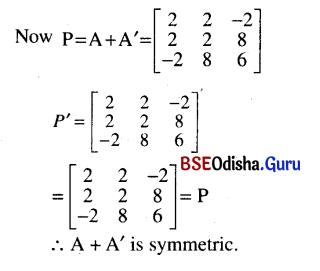(ii) A-A’ is skew-symmetric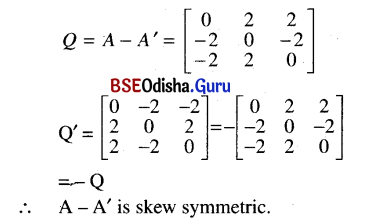Question 6.
Prove that a unit matrix is its own inverse. Is the converse true?
IfA = $$\left[\begin{array}{rrr} 0 & 1 & -1 \\ 4 & -3 & 4 \\ 3 & -3 & 4 \end{array}\right]$$ show that A2 = I and hence A= A-1.
Solution:
No the converse is not true for example: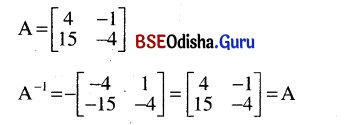Question 7.
Here A is an involuntary matrix, recall the definition given earlier.
Solution: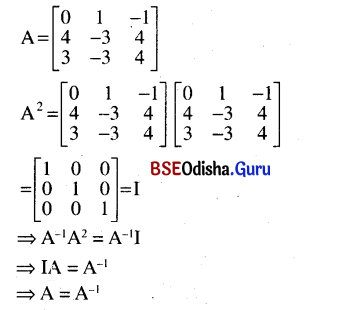Question 8.
Show that $$\left[\begin{array}{ll} \mathbf{0} & \mathbf{1} \\ \mathbf{1} & \mathbf{0} \end{array}\right]$$ is its own inverse.
Solution: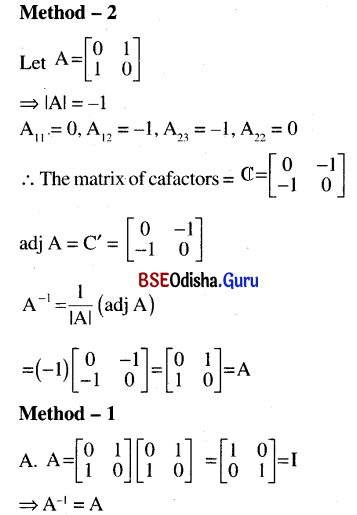Question 9.
Express as a sum of a symmetric and a skew symmetric matrix.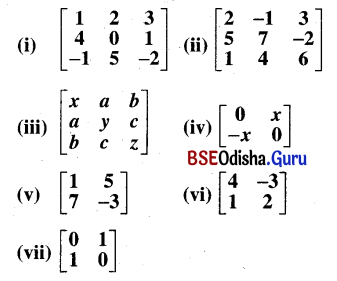Solutions: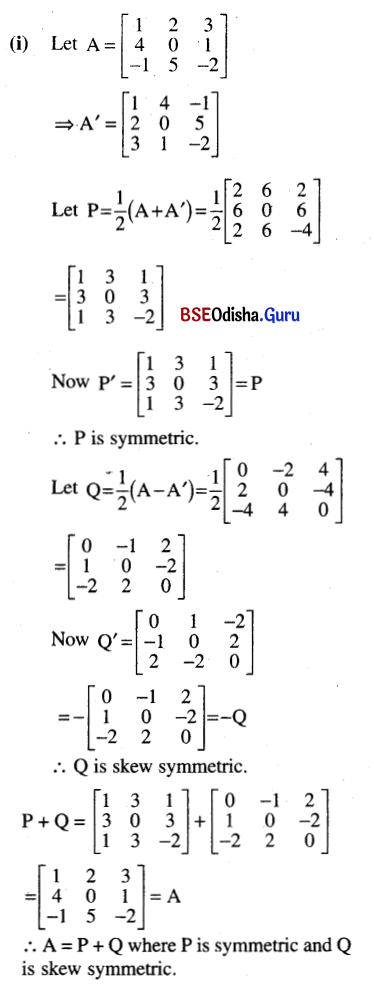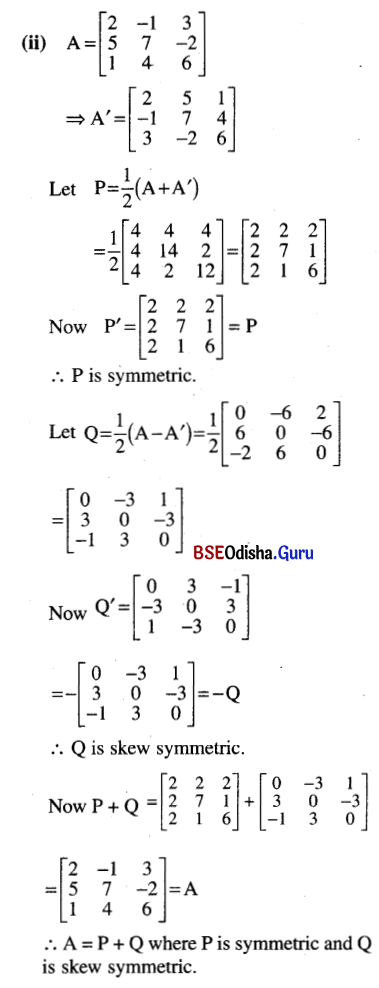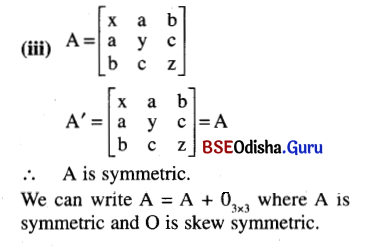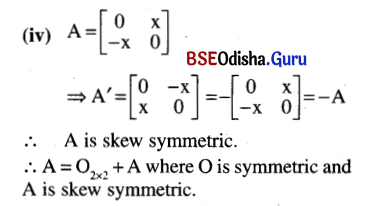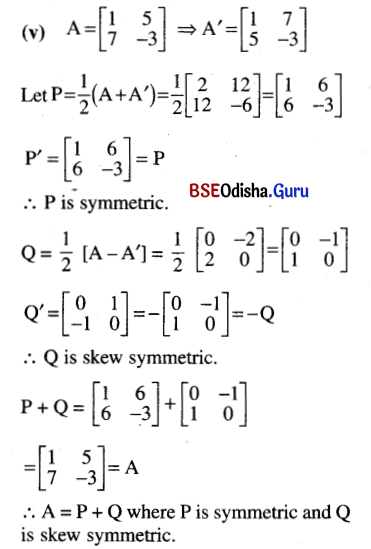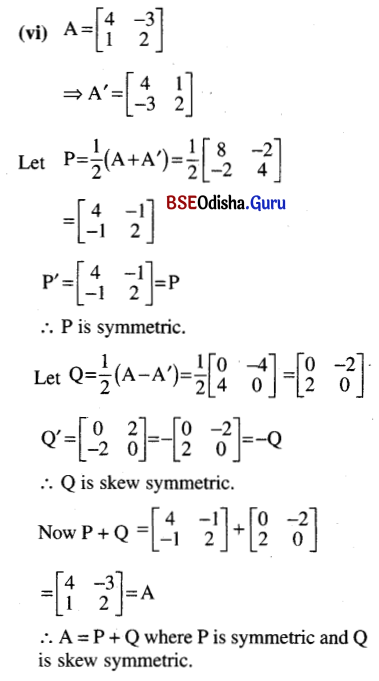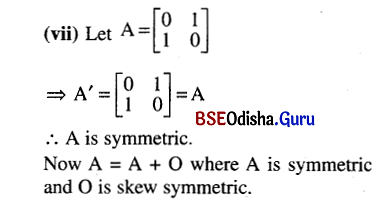Question 10.
What is the inverse of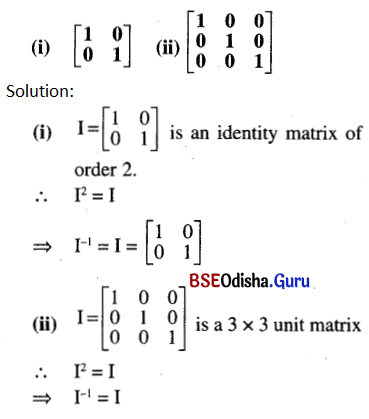Question 11.
Find inverse of the following matrices by elementary row/column operation (transformations):
(i) $$\left[\begin{array}{ll} 1 & 2 \\ 3 & 5 \end{array}\right]$$
Solution: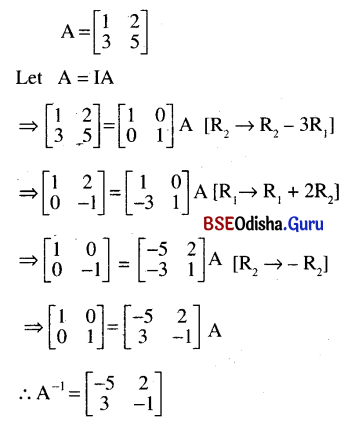(ii) $$\left[\begin{array}{ll} 2 & 5 \\ 1 & 3 \end{array}\right]$$
Solution: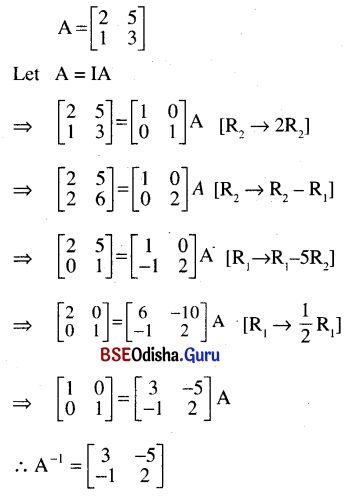(iii) $$\left[\begin{array}{cc} 4 & -2 \\ 3 & 1 \end{array}\right]$$
Solution: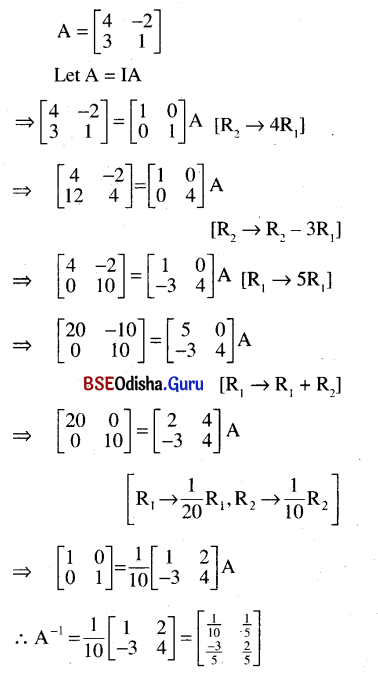(iv) $$\left[\begin{array}{ll} 2 & 5 \\ 1 & 3 \end{array}\right]$$
Solution: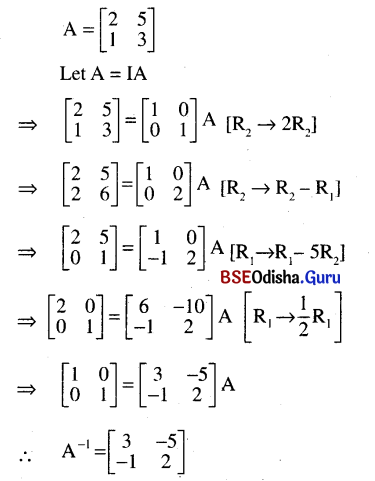(v) $$\left[\begin{array}{cc} 1 & 0 \\ 2 & -3 \end{array}\right]$$
Solution: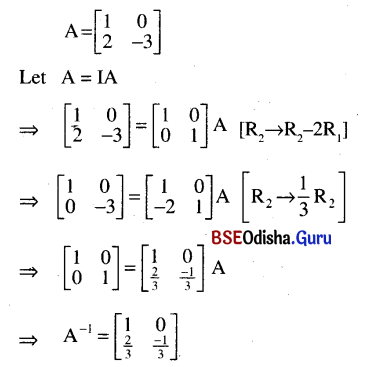(vi) $$\left[\begin{array}{cc} 1 & 0 \\ 0 & -1 \end{array}\right]$$
Solution: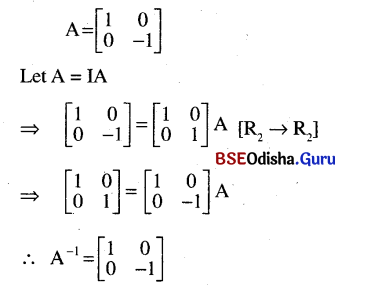Question 12.
Find the inverse of the following matrices using elementary transformation:
(i) $$\left[\begin{array}{lll} \mathbf{0} & \mathbf{0} & 2 \\ \mathbf{0} & \mathbf{2} & \mathbf{0} \\ \mathbf{2} & \mathbf{0} & \mathbf{0} \end{array}\right]$$
Solution: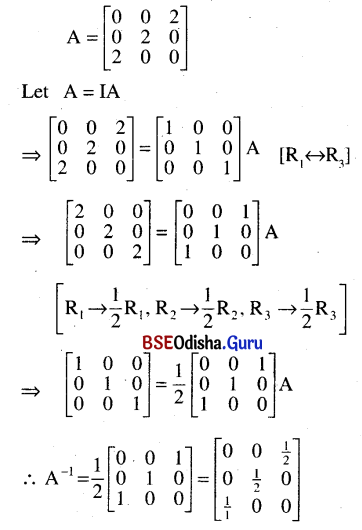(ii) $$\left[\begin{array}{lll} 0 & 1 & 2 \\ 1 & 2 & 3 \\ 3 & 1 & 1 \end{array}\right]$$
Solution: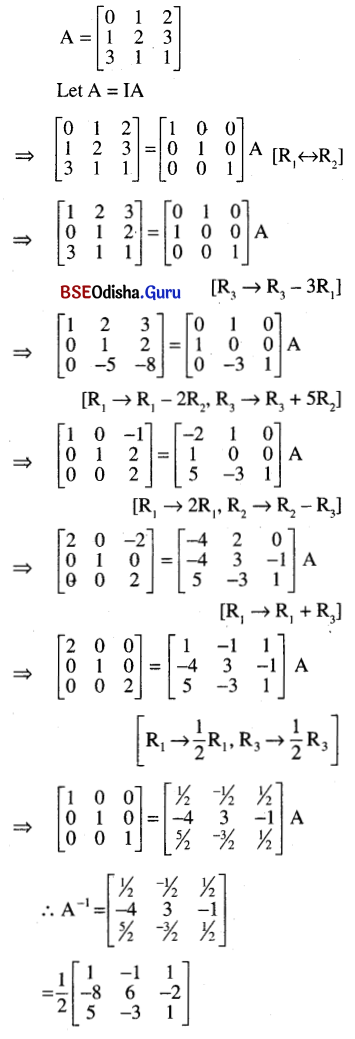(iii) $$\left[\begin{array}{ccc} 3 & -2 & 3 \\ 2 & 1 & -1 \\ 4 & -3 & 2 \end{array}\right]$$
Solution: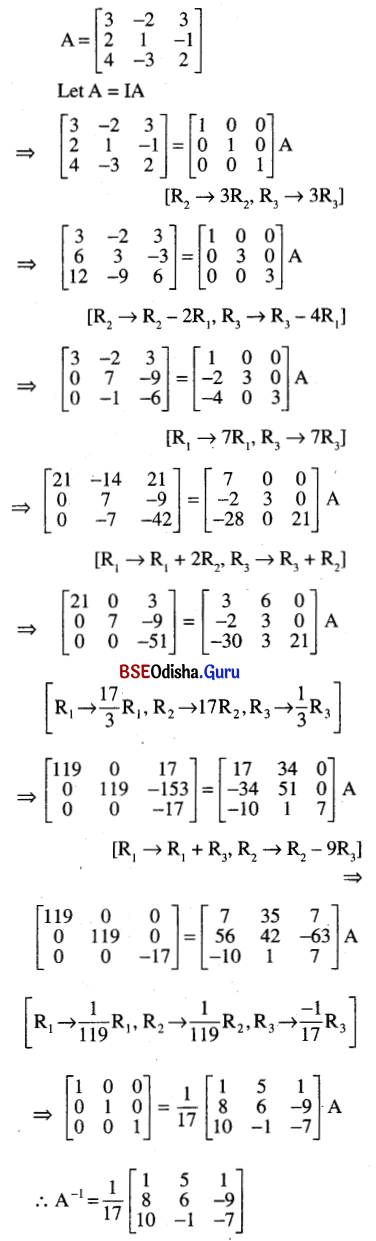(iv) $$\left[\begin{array}{lll} 1 & 1 & 2 \\ 0 & 1 & 2 \\ 1 & 2 & 1 \end{array}\right]$$
Solution: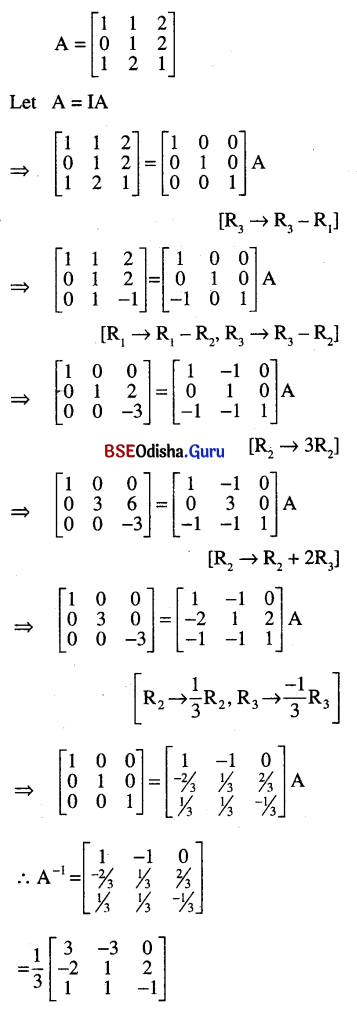(v) $$\left[\begin{array}{lll} 1 & 2 & 3 \\ 2 & 1 & 4 \\ 1 & 0 & 2 \end{array}\right]$$
Solution: